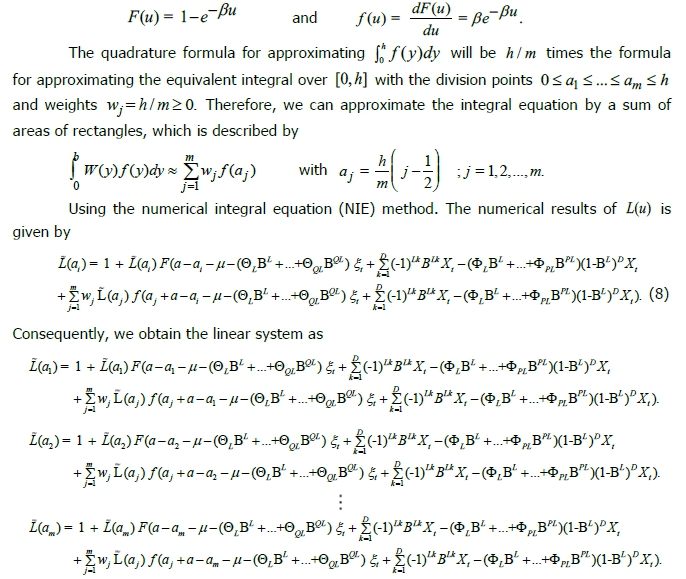# Estimating the Average Run Length of CUSUM Control Chart for Seasonal Autoregressive Integrated Moving Average of Order (P,D,Q)L Model

• Suvimol Phanyaem King Mongkut's University of Technology North Bangkok
Keywords: Average Run Length, Cumulative Sum Control Chart, Seasonal Autoregressive Integrated Moving Average

### Abstract

The main objective of this paper is to present the numerical integral equation of average run length (ARL) of cumulative sum (CUSUM) control chart when observations are seasonal autoregressive integrated moving average denoted by SARIMA(P,D,Q)L process with exponential white noise. The accuracy of the proposed method is established by comparing them to the Monte Carlo simulation method in term of the absolute percentage difference and the computational times. A comparison of the results obtained from the numerical integral equation with the results obtained from the Monte Carlo simulation shows that the absolute percentage difference is less than 1.0%. In terms of computational time, we find that the computational times are approximately 60 minute for the numerical integration method and approximately 190 min for the Monte Carlo simulation method.